# List of Mathematical Functions and Expressions in MATLAB | Example ExplainedWe all know, MATLAB is used for the mathematical calculation like as a calculator. It is also used for technical computing. And there are different mathematical functions and expressions in MATLAB for computing.

I remember, when I was solving the mathematical equations in MATLAB, I was frantically looking for the answer of a couple questions.

1. What is the syntax for performing the mathematical calculation in MATLAB?
2. How to write the mathematical expressions and functions in MATLAB?

Here,

In this tutorials, I am sharing a list of various math functions and their short abbreviations. This will help you for calculation in the MATLAB Window. Also, it will help you solving basic examples with the mathematical expressions.

What is Expression in general?

The expressions consist of the various math functions like as arithmetic, trigonometric, logarithmic, exponential, constant term value, etc. These functions have proper syntax.

So, you must know the syntax of the mathematical functions. Without this, it will not be easy to solve the problems in MATLAB.

Let’s see the below syntax for mathematical functions.

### Mathematical Functions and Expressions in MATLAB with Syntax

Here is different Mathematical functions and their syntax for MATLAB.

• Arithmetic functions
 Arithmetic Symbol Operation + Addition – Subtraction * Multiplication / Division
• Trigonometric functions
 Trigonometric Symbol Operation / Function sin(t) Performs Sin operation on variable ‘t’. cos(t) Performs cosine operation on variable ‘t’. tan(t) Performs tangent operation on variable ‘t’. asin(t) Performs arc sin operation on variable ‘t’ or Inverse of the sin function. acos(t) Performs arc cosine operation on variable ‘t’ or Inverse of the cos function. atan(t) Performs arc tangent operation on variable ‘t’ or Inverse of the tan function.
• Exponential functions
 Exponetial Symbol Operation exp(t) Performs exponential operation on variable ‘t’.
• Square functions
 Symbol Operation ^ Power or Square sqrt(t) Performs square root operation on variable ‘t’.
• Logarithm functions
 Symbol Operation log(t) Performs a natural logarithmic operation on variable ‘t’. log10(t) Performs a common logarithmic operation on variable ‘t’.
• Maximum & Minimum functions
 Symbol Operation min(t) Finds minimum value from array ‘t’. max(t) Finds maximum value from array ‘t’.
• Remainder function
 Symbol Operation rem(p,q) Gives the remainder after the dividing ‘p’ by ‘q’.
• Phase angle function
 Symbol Operation angel(t) Gives phase angle for variable ‘t’.
• Other useful functions
 Symbol Operation abs(t) Returns absolute value for variable ‘t’. sign(t) Returns sign of the variable ‘t’. ceil(t) Returns ceil value for variable ‘t’. floor(t) Returns floor value for variable ‘t’. conj(t) Gives complex conjugate of variable ‘t’. round(t) Returns nearest integer of variable ‘t’.
• Constant term value functions
 Symbol / Constant Associated Constant value pi The ‘π’ number = 3.14159… i, j The imaginary unit √-1 Inf The infinity, ∞

These are the mathematical functions representation in the MATLAB.

### How to write the Mathematical Expression in MATLAB?

Let’s do this by solving some basic problems in the MATLAB software.

Some general mathematical equations are…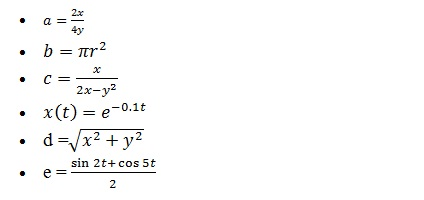#### Example 1: How to calculate the value of ‘a’ for below example?

`a = (2*x)/(4*y)`

Solution: Suppose, the value of variable ‘x’,  and ‘y’ are 12 and 10, respectively. Put these two values in the above equation and calculate the value of the variable ‘a’.

Output in MATLAB:

```>> x=12; y=10;

>> a=(2*x)/(4*y)

a     =
0.6000```

Screenshot of the expression in the MATLAB window.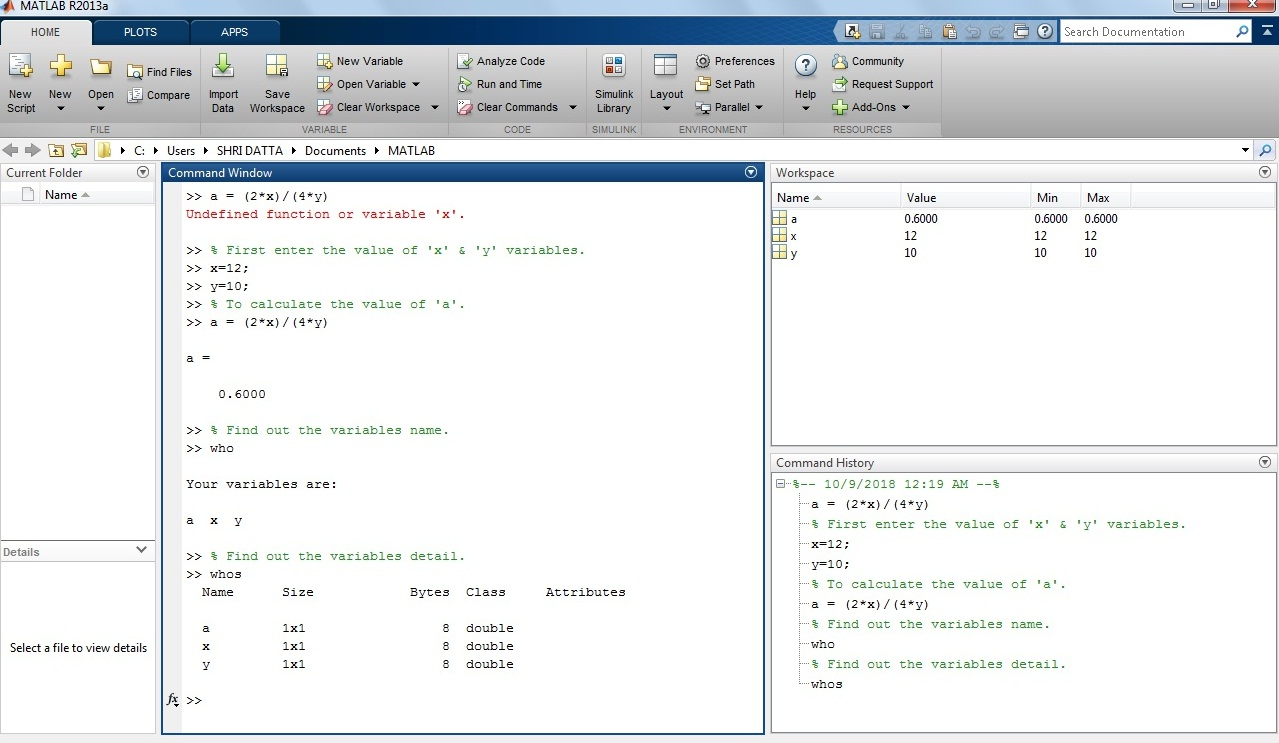What is who and whos in MATLAB?

If you look at the above screenshot, I am using ‘who’ and ‘whos’ keyword. The keyword ‘who’ gives the list of the variable used. The keyword ‘whos’ shows detail like name, size, class and attributes for each variable.

#### Example 2: How to calculate the area of a circle in MATLAB?

`b = pi*(r^2)`

Solution: Take the value of radius ‘r’ variable 4. Put this value in the equation.

Output in MATLAB:

```>> r=4;

>> b= pi*(r^2)

b    =
50.2655```

Screenshot of the expression in the MATLAB window for calculating the area of a circle.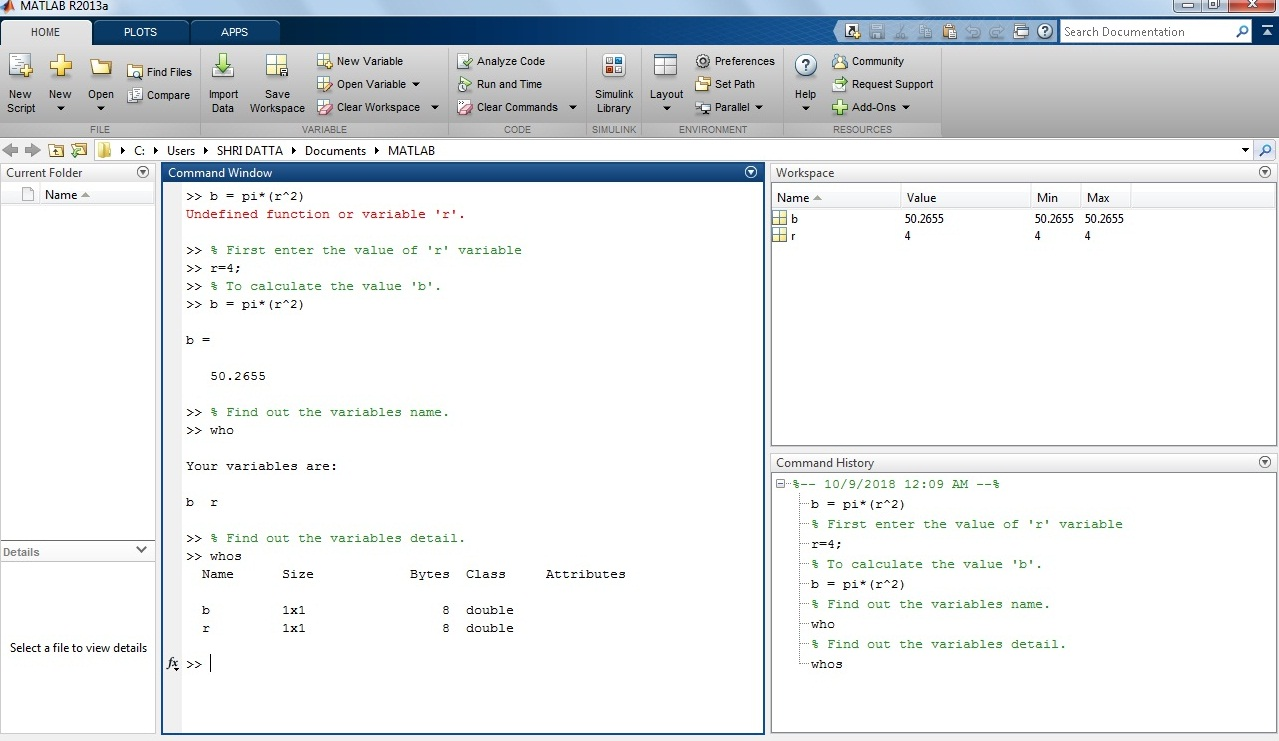#### Example 3: How to calculate the value of ‘c’ for below expression?

`c = (x/2)*((x-y)^2)`

Solution: Consider, variable value of ‘x’ and ‘y’ are 1 and 5 respectively.

Output in MATLAB:

```>> x=1; y=5;

>> c=(x/2)*((x-y)^2)

c    =
8```

Screenshot of the expression in the MATLAB window.#### Example 4: How to calculate the exponential value in MATLAB?

• `x(t) = exp (-0.1*t)`

Solution: Let the value of  ‘t’ variables is 2,

Suppose,

```>> t=2;

>> x(t)= exp(-0.1*t)

x(t)    =
81.87```

Screenshot of the expression in the MATLAB window.#### Example 5: How to calculate square root expression in MATLAB?

`d = sqrt((x^2)+(y^2))`

Solution: In the above equation, put the value of ‘x’ and ‘y’ variables 13 and 8 respectively.

```>> x=13; y=8;

>> d= sqrt((x^2)+(y^2))

d    =
15.2643```

Screenshot of the square expression in the MATLAB window.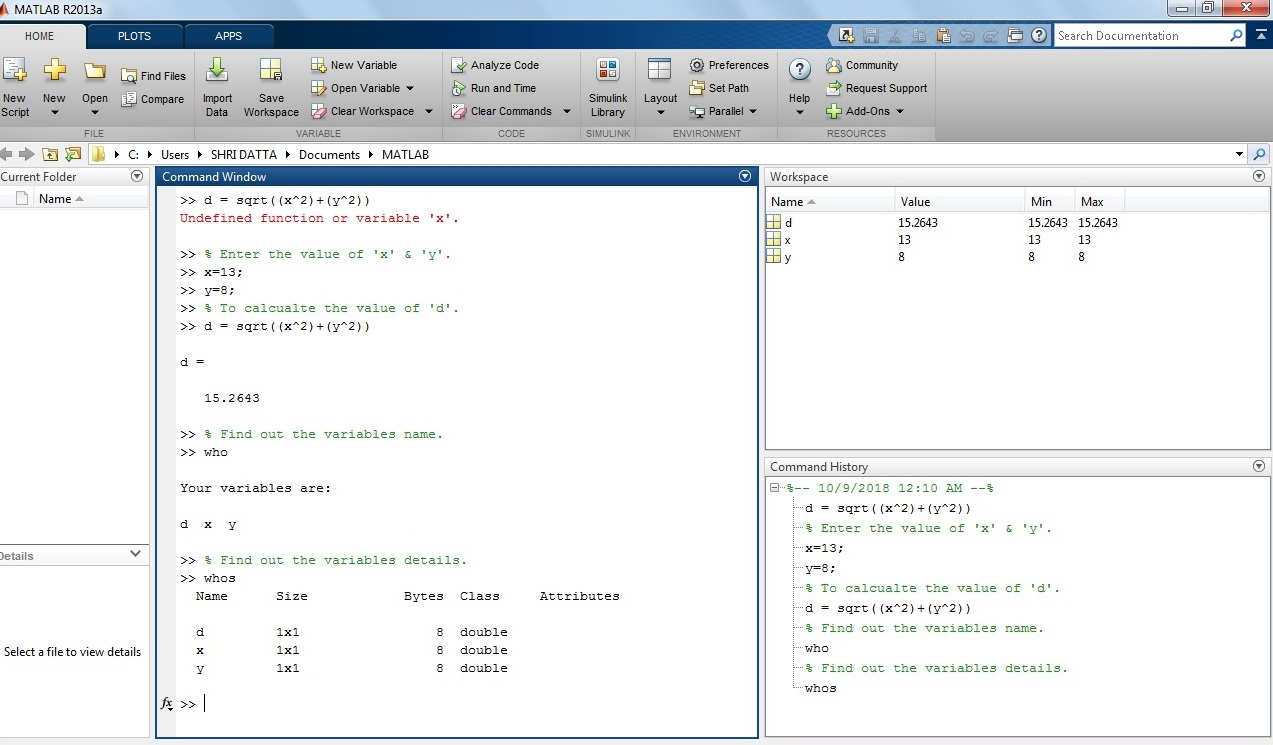#### Example 6: How to calculate trigonometric expression in MATLAB?

`e = (sin(2*t) + cos(5*t)) /2`

Solution: Put the value of ‘t’ variable in the above equation.

Let’s take t = 0.1.

```>>t=0.1 ;

>> e= (sin (2*t)+cos(5*t))/2

e   =
0.5381```

Screenshot of the trigonometric expression in the MATLAB window.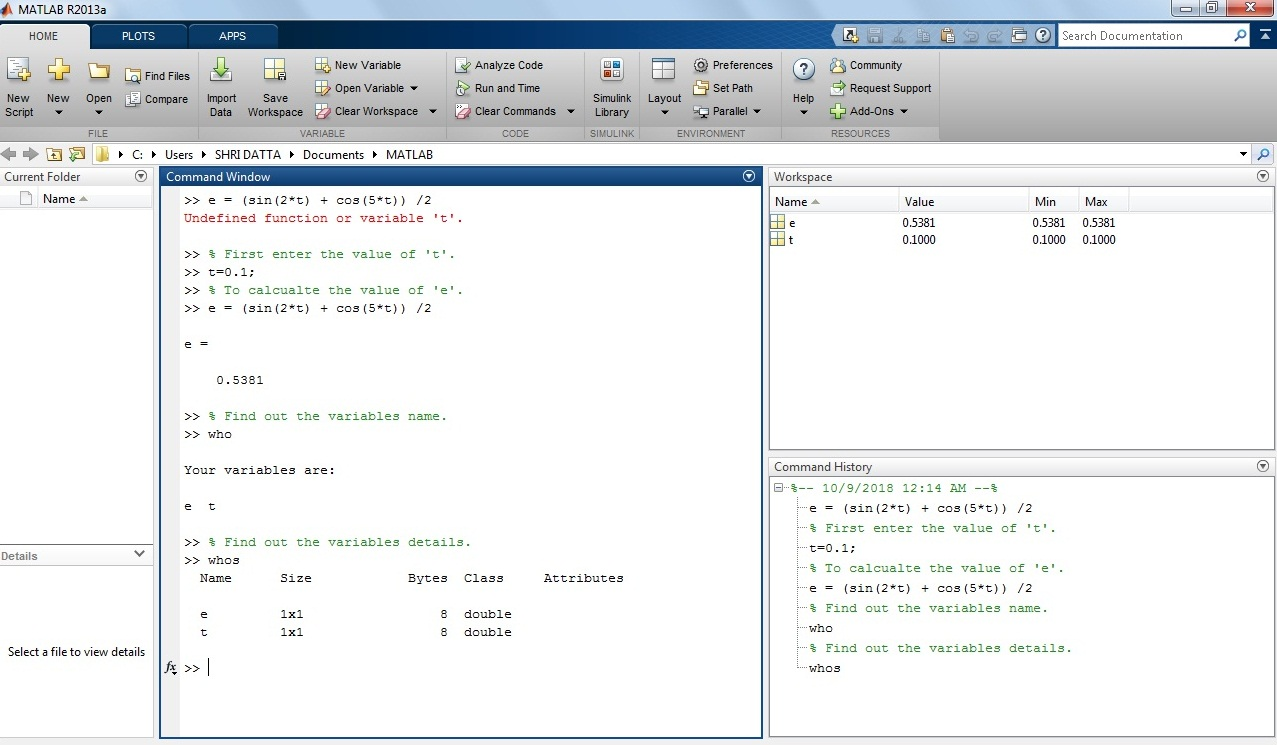In this tutorial, you will find all basic syntax for mathematical functions and expressions in MATLAB. You need them for the solving problems.

If you have any query related to this topic, let’s discuss this in the comment section below.

If You Appreciate What I Do Here On DipsLab, You Should Consider:

DipsLab is the fastest growing and most trusted community site for Electrical and Electronics Engineers. All the published articles are available FREELY to all.

If you like what you are reading, please consider buying me a coffee ( or 2 ) as a token of appreciation.

This will help me to continue my services and pay the bills.I’m thankful for your never-ending support.

### 2 thoughts on “List of Mathematical Functions and Expressions in MATLAB | Example Explained”

1.Thanks for spending some time to share the article on your website.

•